Orthogonality of the DFT Sinusoids Next  |  Prev  |  Up  |  Top  |  Index  |  JOS Index  |  JOS Pubs  |  JOS Home  |  Search

## Orthogonality of the DFTSinusoids

We now show mathematically that the DFT sinusoids are exactly orthogonal. Let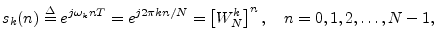denote the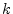th DFT complex-sinusoid, for. Then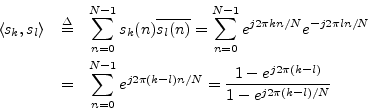where the last step made use of the closed-form expression for the sum of a geometric series6.1). If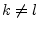, the denominator is nonzero while the numerator is zero. This proves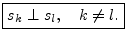While we only looked at unit amplitude, zero-phase complex sinusoids, as used by the DFT, it is readily verified that the (nonzero) amplitude and phase have no effect on orthogonality.

Next  |  Prev  |  Up  |  Top  |  Index  |  JOS Index  |  JOS Pubs  |  JOS Home  |  Search

[How to cite this work] [Order a printed hardcopy]